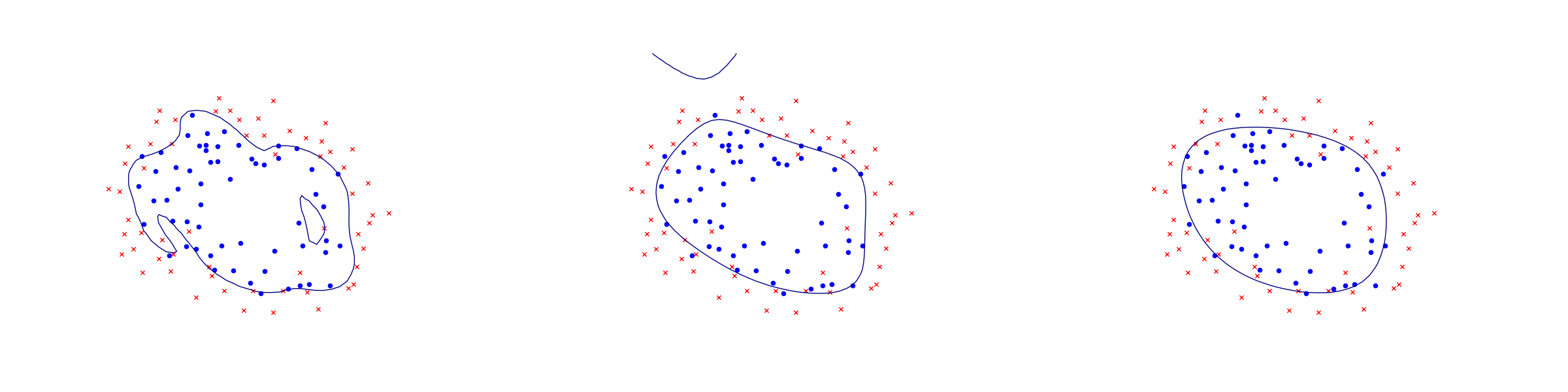# 11.5 Logistic regression¶

Logistic regression is an example of a binary classifier, where the output takes one two values 0 or 1 for each data point. We call the two values classes.

Formulation as an optimization problem

Define the sigmoid function

$S(x)=\frac{1}{1+\exp(-x)}.$

Next, given an observation $$x\in\real^d$$ and a weights $$\theta\in\real^d$$ we set

$h_\theta(x)=S(\theta^Tx)=\frac{1}{1+\exp(-\theta^Tx)}.$

The weights vector $$\theta$$ is part of the setup of the classifier. The expression $$h_\theta(x)$$ is interpreted as the probability that $$x$$ belongs to class 1. When asked to classify $$x$$ the returned answer is

$\begin{split}x\mapsto \begin{cases}\begin{array}{ll}1 & h_\theta(x)\geq 1/2, \\ 0 & h_\theta(x)<1/2.\end{array}\end{cases}\end{split}$

When training a logistic regression algorithm we are given a sequence of training examples $$x_i$$, each labelled with its class $$y_i\in \{0,1\}$$ and we seek to find the weights $$\theta$$ which maximize the likelihood function

$\prod_i h_\theta(x_i)^{y_i}(1-h_\theta(x_i))^{1-y_i}.$

Of course every single $$y_i$$ equals 0 or 1, so just one factor appears in the product for each training data point. By taking logarithms we can define the logistic loss function:

$J(\theta) = -\sum_{i:y_i=1} \log(h_\theta(x_i))-\sum_{i:y_i=0}\log(1-h_\theta(x_i)).$

The training problem with regularization (a standard technique to prevent overfitting) is now equivalent to

$\min_\theta J(\theta) + \lambda\|\theta\|_2.$

This can equivalently be phrased as

(11.20)$\begin{split}\begin{array}{lrllr} \minimize & \sum_i t_i +\lambda r & & & \\ \st & t_i & \geq - \log(h_\theta(x)) & = \log(1+\exp(-\theta^Tx_i)) & \mathrm{if}\ y_i=1, \\ & t_i & \geq - \log(1-h_\theta(x)) & = \log(1+\exp(\theta^Tx_i)) & \mathrm{if}\ y_i=0, \\ & r & \geq \|\theta\|_2. & & \end{array}\end{split}$

Implementation

As can be seen from (11.20) the key point is to implement the softplus bound $$t\geq \log(1+e^u)$$, which is the simplest example of a log-sum-exp constraint for two terms. Here $$t$$ is a scalar variable and $$u$$ will be the affine expression of the form $$\pm \theta^Tx_i$$. This is equivalent to

$\exp(u-t) + \exp(-t)\leq 1$

and further to

(11.21)$\begin{split}\begin{array}{rclr} (z_1, 1, u-t) & \in & \EXP & (z_1\geq \exp(u-t)), \\ (z_2, 1, -t) & \in & \EXP & (z_2\geq \exp(-t)), \\ z_1+z_2 & \leq & 1. & \end{array}\end{split}$
Listing 11.11 Implementation of $$t\geq \log(1+e^u)$$ as in (11.21). Click here to download.
  // t >= log( 1 + exp(u) ) coordinatewise
public static void softplus(Model      M,
Expression t,
Expression u)
{
int n = t.getShape();
Variable z1 = M.variable(n);
Variable z2 = M.variable(n);
M.constraint(Expr.hstack(z1, Expr.constTerm(n, 1.0), Expr.sub(u,t)), Domain.inPExpCone());
M.constraint(Expr.hstack(z2, Expr.constTerm(n, 1.0), Expr.neg(t)), Domain.inPExpCone());
}


Once we have this subroutine, it is easy to implement a function that builds the regularized loss function model (11.20).

Listing 11.12 Implementation of (11.20). Click here to download.
  // Model logistic regression (regularized with full 2-norm of theta)
// X - n x d matrix of data points
// y - length n vector classifying training points
// lamb - regularization parameter
public static Model logisticRegression(double[][] X,
boolean[]  y,
double     lamb)
{
int n = X.length;
int d = X.length;       // num samples, dimension

Model M = new Model();

Variable theta = M.variable("theta", d);
Variable t     = M.variable(n);
Variable reg   = M.variable();

M.constraint(Var.vstack(reg, theta), Domain.inQCone());

double[] signs = new double[n];
for(int i = 0; i < n; i++)
if (y[i]) signs[i] = -1; else signs[i] = 1;

softplus(M, t, Expr.mulElm(Expr.mul(X, theta), signs));

return M;
}


Example: 2D dataset fitting

In the next figure we apply logistic regression to the training set of 2D points taken from the example ex2data2.txt . The two-dimensional dataset was converted into a feature vector $$x\in\real^{28}$$ using monomial coordinates of degrees at most 6.Fig. 11.6 Logistic regression example with none, medium and strong regularization (small, medium, large $$\lambda$$). Without regularization we get obvious overfitting.¶